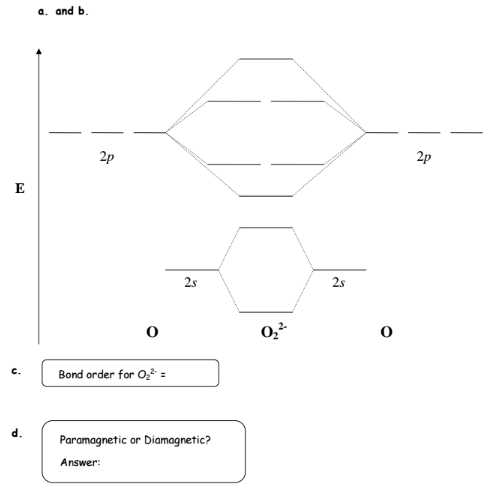# Problem: Complete the MO energy level diagram for O22-. a. Fill in the electrons using arrows (↑ and/or ↓) for the atomic and molecular orbitals. b. Designate all the energy levels (i.e., σ2s*, π2p, σ2s, etc.)  c. Calculate the bond order for O22-. d. Is O2 2- a paramagnetic or diamagnetic chemical species?

###### FREE Expert Solution
91% (359 ratings)
###### Problem Details

Complete the MO energy level diagram for O22-.

a. Fill in the electrons using arrows (↑ and/or ↓) for the atomic and molecular orbitals.

b. Designate all the energy levels (i.e., σ2s*, π2p, σ2s, etc.)

c. Calculate the bond order for O22-.

d. Is O2 2- a paramagnetic or diamagnetic chemical species?What scientific concept do you need to know in order to solve this problem?

Our tutors have indicated that to solve this problem you will need to apply the Bond Order concept. If you need more Bond Order practice, you can also practice Bond Order practice problems.

What is the difficulty of this problem?

Our tutors rated the difficulty ofComplete the MO energy level diagram for O22-. a. Fill in t...as high difficulty.

How long does this problem take to solve?

Our expert Chemistry tutor, Dasha took 7 minutes and 22 seconds to solve this problem. You can follow their steps in the video explanation above.

What professor is this problem relevant for?

Based on our data, we think this problem is relevant for Professor Chan's class at UCLA.